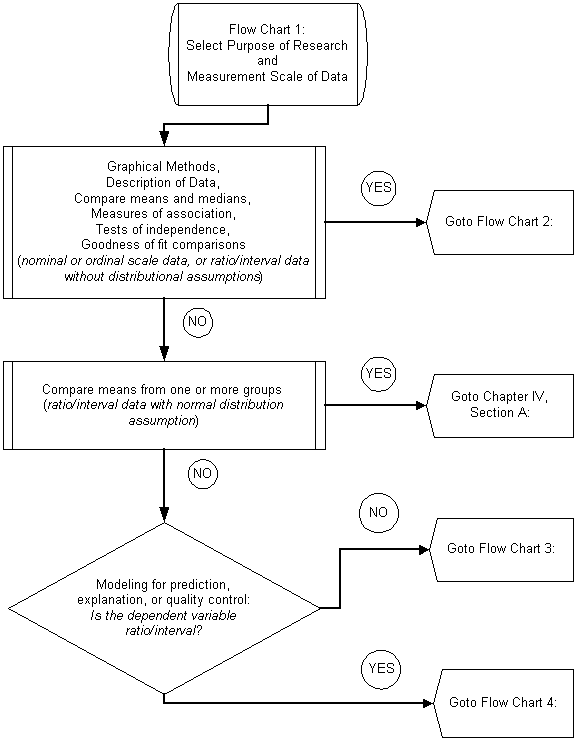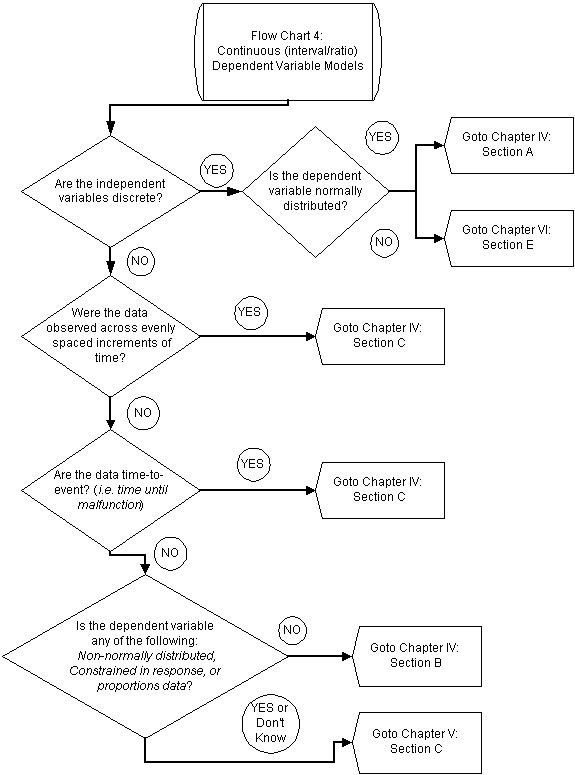Chapter

3

Identification of Appropriate Statistical Method

The purpose of this chapter is to identify the appropriate statistical method(s) for analyzing a specific type of data. The chapter consists largely of flow charts to guide the analyst through a series of questions, eventually directing the analyst to the correct statistical method.

Purpose of this chapter

To identify the appropriate statistical technique for a specific data analysis problem.

Basic Assumptions/Requirements of this chapter

1)       The analyst has designed a research study, identified data needs, and developed statistical hypothesis in which to test,

2)       The analyst can answer questions about the types of variables, both dependent and independent, collected in the study, and

3)       The analyst knows the analysis objective: e.g. modeling, comparison of distributions, test of independence of variables, comparison of distributions parameters, graphical methods, etc.

4)       The analyst must have access to statistical computer software, such that some of the questions regarding data can be answered using graphical methods.

Methodology

The methodology for identifying the appropriate statistical method is as follows. Identify the first single analytical task that must be performed with the data, and then proceed through the flowcharts. Use flowcharts 1 through 4 on the following pages to provide direction to the appropriate chapter and section in the manual. Always begin with flowchart 1, which will lead directly to one of the other flowcharts (2 through 4), which then leads to a technical section in the manual for use in applying the statistical method of choice. Use the flowchart for each analytical task needed for the research.

Flow Chart 1Flow Chart 2Flow Chart 3Flow Chart 4